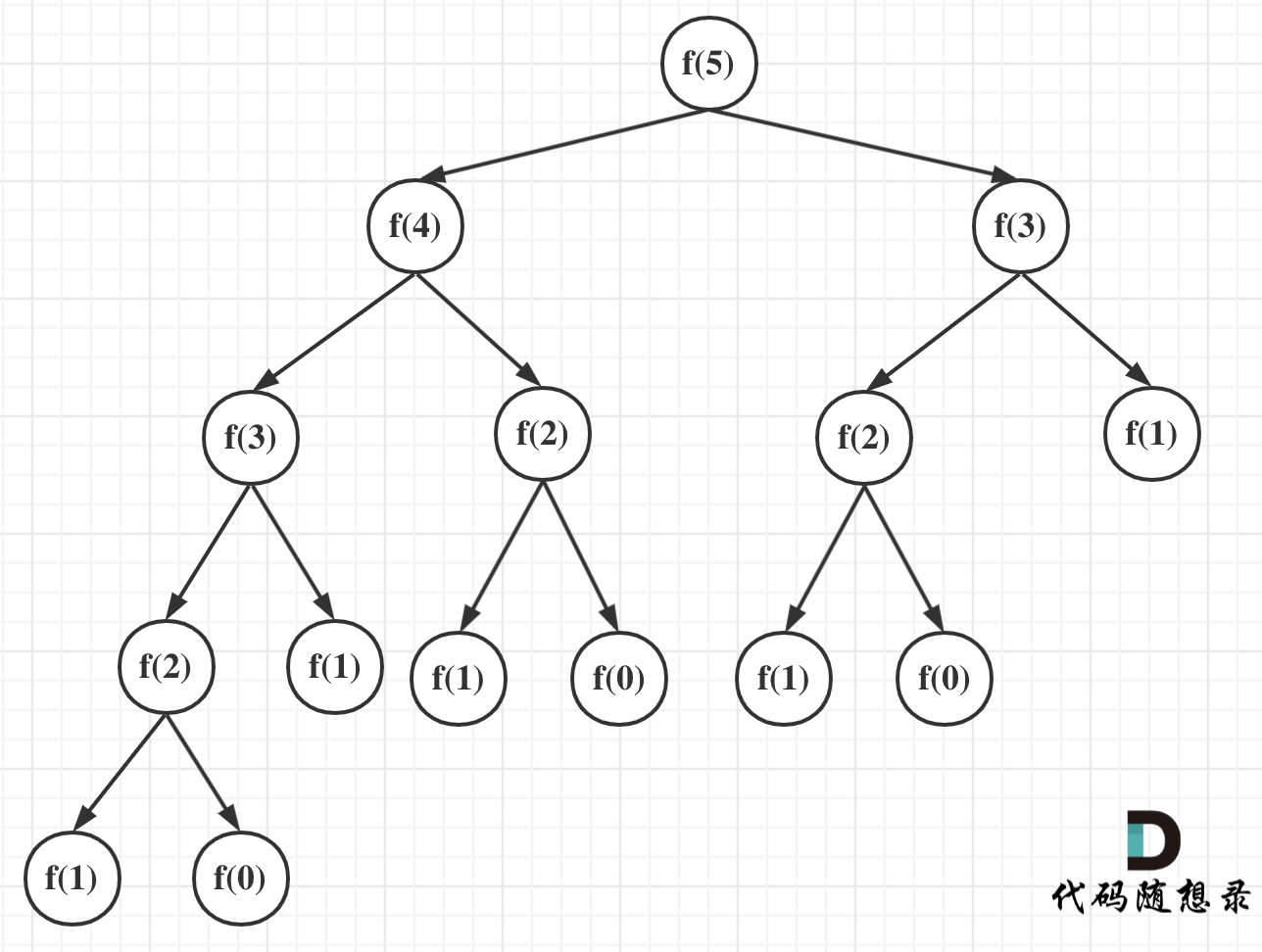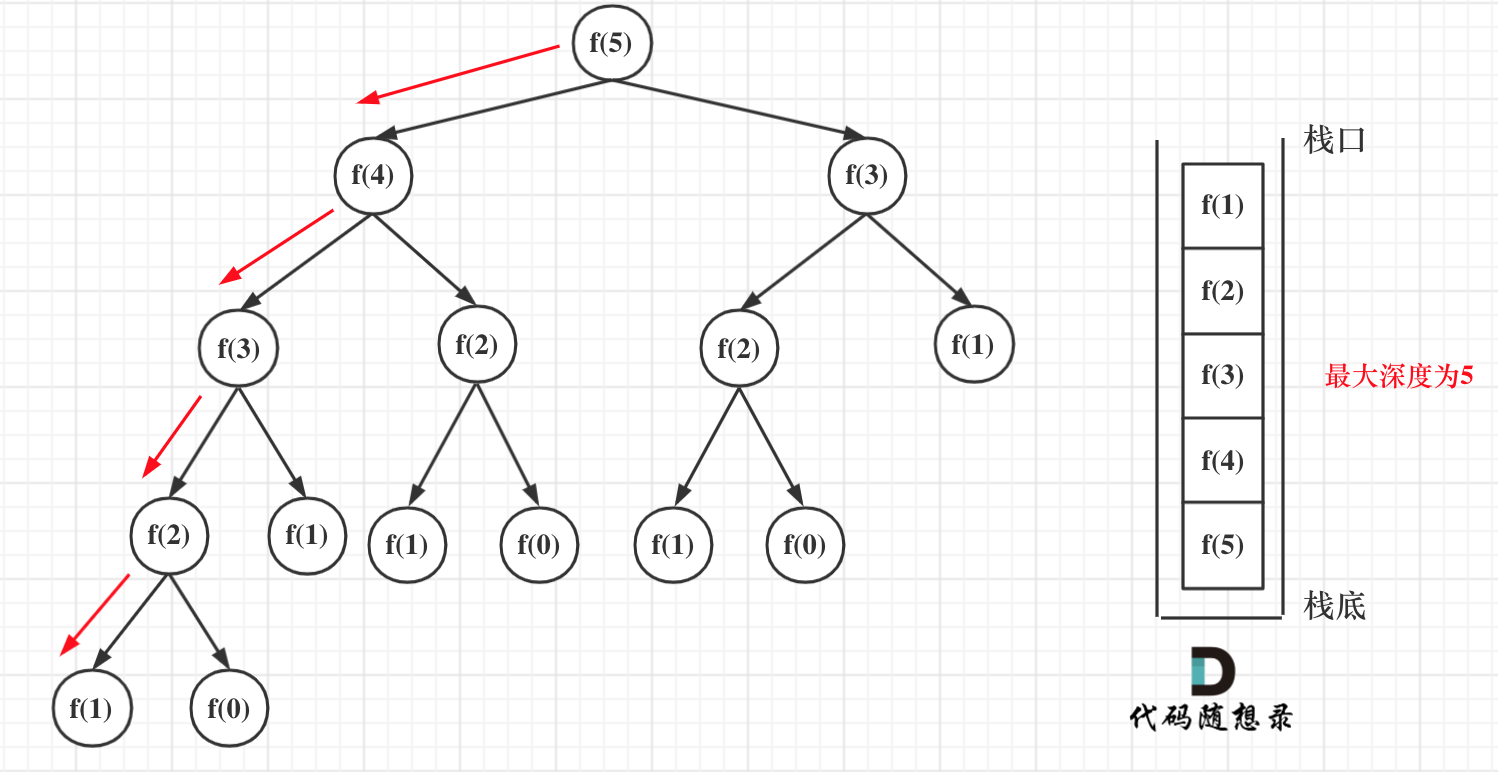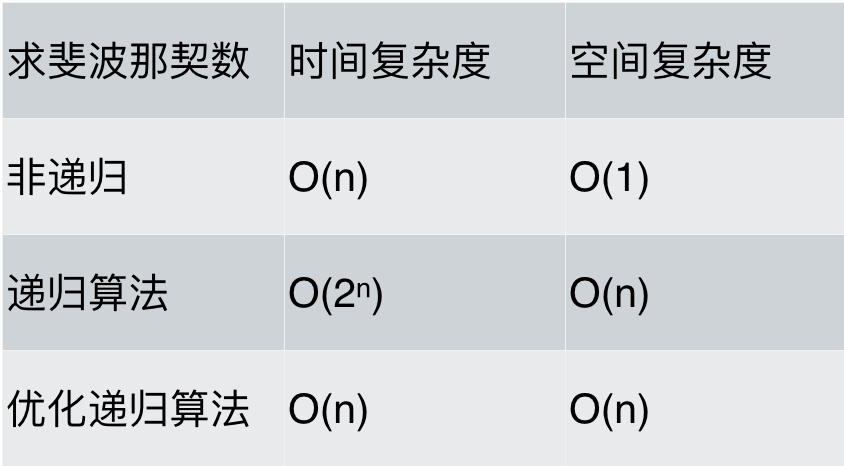# # 递归算法的时间与空间复杂度分析！

## # 递归求斐波那契数列的性能分析

``````int fibonacci(int i) {
if(i <= 0) return 0;
if(i == 1) return 1;
return fibonacci(i-1) + fibonacci(i-2);
}
``````
1
2
3
4
5

### # 时间复杂度分析``````#include <iostream>
#include <chrono>
using namespace std;
using namespace chrono;
int fibonacci(int i) {
if(i <= 0) return 0;
if(i == 1) return 1;
return fibonacci(i - 1) + fibonacci(i - 2);
}
void time_consumption() {
int n;
while (cin >> n) {
milliseconds start_time = duration_cast<milliseconds >(
system_clock::now().time_since_epoch()
);

fibonacci(n);

milliseconds end_time = duration_cast<milliseconds >(
system_clock::now().time_since_epoch()
);
cout << milliseconds(end_time).count() - milliseconds(start_time).count()
<<" ms"<< endl;
}
}
int main()
{
time_consumption();
return 0;
}
``````
1
2
3
4
5
6
7
8
9
10
11
12
13
14
15
16
17
18
19
20
21
22
23
24
25
26
27
28
29
30
31

• n = 40，耗时：837 ms
• n = 50，耗时：110306 ms

``````return fibonacci(i-1) + fibonacci(i-2);
``````
1

``````// 版本二
int fibonacci(int first, int second, int n) {
if (n <= 0) {
return 0;
}
if (n < 3) {
return 1;
}
else if (n == 3) {
return first + second;
}
else {
return fibonacci(second, first + second, n - 1);
}
}
``````
1
2
3
4
5
6
7
8
9
10
11
12
13
14
15

• 时间复杂度： O(n)
• 空间复杂度： O(n)

``````#include <iostream>
#include <chrono>
using namespace std;
using namespace chrono;
int fibonacci_3(int first, int second, int n) {
if (n <= 0) {
return 0;
}
if (n < 3) {
return 1;
}
else if (n == 3) {
return first + second;
}
else {
return fibonacci_3(second, first + second, n - 1);
}
}

void time_consumption() {
int n;
while (cin >> n) {
milliseconds start_time = duration_cast<milliseconds >(
system_clock::now().time_since_epoch()
);

fibonacci_3(1, 1, n);

milliseconds end_time = duration_cast<milliseconds >(
system_clock::now().time_since_epoch()
);
cout << milliseconds(end_time).count() - milliseconds(start_time).count()
<<" ms"<< endl;
}
}
int main()
{
time_consumption();
return 0;
}

``````
1
2
3
4
5
6
7
8
9
10
11
12
13
14
15
16
17
18
19
20
21
22
23
24
25
26
27
28
29
30
31
32
33
34
35
36
37
38
39
40
41
42

• n = 40，耗时：0 ms
• n = 50，耗时：0 ms

### # 空间复杂度分析``````int fibonacci(int i) {
if(i <= 0) return 0;
if(i == 1) return 1;
return fibonacci(i-1) + fibonacci(i-2);
}
``````
1
2
3
4
5### # 二分法（递归实现）的性能分析

``````int binary_search( int arr[], int l, int r, int x) {
if (r >= l) {
int mid = l + (r - l) / 2;
if (arr[mid] == x)
return mid;
if (arr[mid] > x)
return binary_search(arr, l, mid - 1, x);
return binary_search(arr, mid + 1, r, x);
}
return -1;
}
``````
1
2
3
4
5
6
7
8
9
10
11